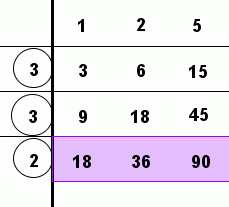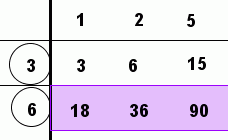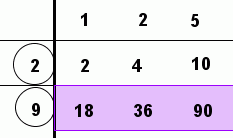﻿ Finding the Greatest Common Factor of 2 or 3 Numbers
FINDING THE GREATEST COMMON FACTOR OF $\,2\,$ or $\,3\,$ NUMBERS
• PRACTICE (online exercises and printable worksheets)
Want more details, more exercises? Read the full text!

EXAMPLE:
Question: Find the greatest common factor of $\,27\,$ and $\,18\,$:
Answer: $\,9$
The idea:
 The factors of $\,27\,$ are: $\,1\,$ $\,3\,$ $\,9\,$ $\,27$ The factors of $\,18\,$ are: $\,1\,$ $\,2\,$ $\,3\,$ $\,6\,$ $\,9\,$ $\,18\,$

The common factors of $\,27\,$ and $\,18\,$ (the numbers that appear in both lists) are $\,1\,$, $\,3\,$, and $\,9\,$.
The greatest common factor (the greatest number in the list of common factors) is $\,9\,$.

EFFICIENT ALGORITHM FOR FINDING THE GREATEST COMMON FACTOR

Here's an efficient algorithm for finding the greatest common factor,
when there aren't too many numbers, and they aren't too big.

The process is illustrated by finding the greatest common factor of $\,18\,$, $\,36\,$, and $\,90\,$:Line up the numbers in a row. (See the purple rectangle at left.) Find ANY number that goes into everything evenly (like $\,2\,$). Do the divisions, and write the results above the original numbers. In the example: $18\,$ divided by $\,2\,$ is $\,9\,$; write the $\,9\,$ above the $\,18\,$ $36\,$ divided by $\,2\,$ is $\,18\,$; write the $\,18\,$ above the $\,36\,$, and so on. Keep repeating the process, until there isn't any number (except $\,1\,$) that goes into everything evenly. Multiply the circled numbers together. This is the greatest common factor! In the example, $\,\text{gcf}(18,36,90) = 2\cdot 3\cdot 3 = 18\,$.
WHY DOES THIS METHOD WORK?

Think about why this method works.
As you walk through each step of this discussion, keep comparing with the chart above.
Look at the prime factorizations of each number:

$18 = 2\cdot3\cdot 3$
$36 = 2\cdot 2\cdot 3\cdot 3$
$90 = 2\cdot3\cdot 3\cdot 5$
The number $\,2\,$ goes into each evenly, so separate it off:
$18 = 2\cdot (3\cdot 3)\hphantom{\cdot 3} = 2\cdot 9$
$36 = 2\cdot (2\cdot 3\cdot 3) = 2\cdot 18$
$90 = 2\cdot (3\cdot 3\cdot 5) = 2\cdot 45$
The number $\,3\,$ goes into each remaining part evenly, so separate it off:
$18 = (2\cdot 3) \cdot (3) \hphantom{\cdot 3}= (2\cdot 3)\cdot 3$
$36 = (2\cdot 3) \cdot (2\cdot 3) = (2\cdot 3)\cdot 6$
$90 = (2\cdot 3) \cdot (3\cdot 5) = (2\cdot 3)\cdot 15$
Here's the final step:
$18 = (2\cdot 3\cdot 3)\cdot 1$
$36 = (2\cdot 3\cdot 3)\cdot 2$
$90 = (2\cdot 3\cdot 3)\cdot 5$
OTHER WAYS TO APPLY THE ALGORITHM

Here are some other ways the algorithm might be applied.
Of course, you get the same answer any correct way that you do it!You can also zip over to wolframalpha.com and type in:   gcd(18,36,90)
The ‘gcd’ stands for greatest common divisor, which is another name for greatest common factor.

BONUS: AN EFFICIENT ALGORITHM FOR FINDING THE LEAST COMMON MULTIPLE

The method described here for efficiently finding the greatest common factor
also gives an efficient way to find the least common multiple (lcm)!
Here's how:

Suppose we now want the least common multiple of $\,18\,$, $\,36\,$, and $\,90\,$.
Start by doing all the same work as above—which is repeated below, for your convenience.

To be a multiple of all three numbers, we first need the shared (common) factors.
These are the numbers in parentheses on the left below, or the vertical (circled) numbers on the right.

To be a multiple of all three numbers, we also need the non-shared factors.
These are the numbers after the parentheses on the left below, or the numbers along the top on the right.

Multiply the shared and non-shared factors together to get the least common multiple!

 $18 = (\color{green}{2\cdot 3\cdot 3})\cdot \color{red}{1}$ $36 = (\color{green}{2\cdot 3\cdot 3})\cdot \color{red}{2}$ $90 = (\color{green}{2\cdot 3\cdot 3})\cdot \color{red}{5}$shared factors in parentheses:   $2\cdot 3\cdot 3$ non-shared factors after parentheses:   $1\cdot 2\cdot 5$ \begin{align} \text{lcm} &= (\text{shared})(\text{non-shared})\cr &= (2\cdot 3\cdot 3)\cdot (1\cdot 2\cdot 5)\cr &= 180 \end{align} shared factors are vertical (circled):   $2\cdot 3\cdot 3$ non-shared factors on the top:   $1\cdot 2\cdot 5$ \begin{align} \text{lcm} &= (\text{shared})(\text{non-shared})\cr &= (2\cdot 3\cdot 3)\cdot (1\cdot 2\cdot 5)\cr &= 180 \end{align}

Want an even more efficient and reliable method?
Zip to WolframAlpha and type in:

least common multiple of 18, 36, 90             or             lcm(18,36,90)
Voila!

Master the ideas from this section